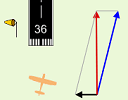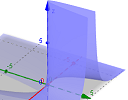Search IntMath
Close

450+ Math Lessons written by Math Professors and Teachers

5 Million+ Students Helped Each Year

1200+ Articles Written by Math Educators and Enthusiasts

Simplifying and Teaching Math for Over 23 Years

# IntMath Newsletter: Vectors activity, GeoGebra 5

By Murray Bourne, 28 Nov 2014

28 Nov 2014

1. Math in cross-wind landing vectors applet
2. GeoGebra 5 now with 3-D graphs
3. Math puzzles
4. Final thought - thank you

## 1. Math in cross-wind landing vectors applet

I recently updated the crosswind landing applet in the Vectors chapter, so it now runs on tablets.There is some interesting math behind this cross wind landing applet. Let's take a look at it: Math in cross-wind landing vectors applet

## 2. GeoGebra 5 now with 3-D graphs

GeoGebra is a great tool for exploring math concepts in a visual way. It is a powerful free graphing tool that allows you to develop your own interactive math applets, with no coding knowledge required (well, a little helps!).

I've been looking forward to the release of version 5 for some time, as it promised 3D graphics. It came out a few months ago and I've been having a play with it recently.

One of the early drawbacks of GeoGebra was that it worked on desktops only (being Java-based). But now it can be used on iOS, Android and Windows tablets, and as for phones, they say it's "coming soon".GeoGebra's latest version offers 3D graphs and output that can be viewed on tablet devices. Here's a quick overview with some graph examples. GeoGebra now with 3D graphs

## 3. Math puzzles

The puzzle in the last IntMath Newsletter asked about cup capacity when mixing gasoline and oil. The correct answer with explanation was given by Chris, Rajat and Nicos.

New math puzzle: What is the value of eln x? How does it differ from the value of ln ex?

(Note: "ln" is the natural logarithm, and "ln x" means "logex". I've always thought the notation for natural logarithm is terrible, and I complain about it when introducing natural logs.)

## 4. Final thought - thank you

Yesterday was Thanksgiving in the USA. In line with this topic, one of my most popular recent tweets was:Education is a wonderful blessing, but sadly, it often seems to be appreciated only by those who don't have easy access to it.

Until next time, enjoy whatever you learn.

1. Doug Gerrard says:

Canadian Thanksgiving: the 2nd Monday in October.
You probably made the mistake to elicit feedback.

2. Miguel Angel Velo says:

The two values are the same. If y=ln(e^x) then e^y=e^x (this is the definition of ln) and y=x. In the other proposition y=x.

3. Guido says:

e^x and ln x are inverse operations. They undo each other,

So e^ln x , e is undoing ln, and you get e^ln x = x.

Similarly, ln (e^x) = x again.

But since the function lnx does not exists for 0 and negative values of x,

e^lnx is only valid for x: }0;+inf},

as for ln (e^x) x is allowed to be negative.

e^x will always be positive.

except : lim e^x for x--->-inf the result will be 0.

Therefore ln (e^x) will be valid for x }-inf;+inf}.

4. Michael London says:

Dear Murray

Thank you for your letter. What I would like to know if you are interested in Wind Energy, and the mathematics behind this topic, which is really highly relevant for the world of today.

Not just wind energy but solar power and the mathematics of solar cells.

5. Murray says:

Yes, Michael, I'm certainly interested in wind energy and sustainable energy generally. I hope to write some articles on this soon!

e^(lnx) = x as the e^x and ln(x) are inverse of each other .
proof :- let take logarithm both side

ln(e^ln(x) ) = ln(x) [:. as e^(lnx) = x ]
=> ln(x) . ln(e) = ln (x) [:. by logarithm properties ]
hence , ln(x) = ln (x) [l.h.s = r.h.s]

It is not much differ from ln (e^x) as both comply on the rule for each others inverse .

7. Michael El-Jiz says:

e^(ln(x)) = x for x>0
x has to be positive for ln(x) to exist. Therefore e^(ln(x)) is undefined for x<=0

ln(e^x) = x
e^x is always positive, therefore ln(e^x) is defined for any x.

8. Nicos Mavrommatis says:

Let ln(e^x)=y. That means: e^y=e^x, so y=x and ln(e^x)=x (1).
From (1): ln[ln(e^x)]=ln(x). Then, e^[ln(x)]=ln(e^x)=x.
The difference is that the function f(x)=e^[ln(x)] has its domain strictly within the 1st quarter of its graph, because there are no logarithms for negative Real numbers.
There is no such a restriction in g(x)=ln(e^x) where x can be negative.
Thus, the two functions are equal only when x has positive entries: f(x)=g(x), xER+

9. Gerard Grufferty says:

The answer is 1 in both cases.

I usually suggest to students that it is like digging a hole today.
Tomorrow fill it up again.

Alternately take off your sock and then put it on again.

e and ln are inverse functions.
Just like square and square root.

On most calculators both functions are on one key.

Good luck with that.

Gerry

10. hamid says:

e^(lnx)=ln(e^x)=x
domain(e^(lnx))=(0,+inf)
domain(ln(e^x))= real numbers
cnclusion:
domain for both functions is diffrent so above functions are not equal.

11. Murray says:

@Doug: Thanks! I've amended the post.

12. Francis Kisner says:

y = e^ln(x) defines the line y = x in the first quadrant.
y = ln(e^x) defines the line y = x in the first and third.

13. Michael Wilde says:

I've downloaded GeoGebra and find it fascinating. But, being a novice at such things as algebra, geometry, and the like, I'll just have to delve more deeply into what the program has to offer and, be assured, it's going to take me quite a while.

14. Murray says:

That's great. Mike! You may find the thousands of existing GeoGebra documents in GeoGebra Tube useful.

15. Francisco Gudiño says:

Dear Mr Bourne.
In e^ln(x)let ln(x)=t.By definition e^t=x.
Thus e^ln(x)=e^t so e^ln(x)=x.

In ln(e^x)let e^x=t.
Then ln(e^x)=ln(t)
Let now ln(t)=u.By definition e^u=t.
Then e^x=e^u;implies u=x.
Therefore ln(e^x)=ln(t)=u=x
So e^ln(x)=ln(e^x).
Best regards.

### Comment Preview

HTML: You can use simple tags like <b>, <a href="...">, etc.

To enter math, you can can either:

1. Use simple calculator-like input in the following format (surround your math in backticks, or qq on tablet or phone):
a^2 = sqrt(b^2 + c^2)
(See more on ASCIIMath syntax); or
2. Use simple LaTeX in the following format. Surround your math with $$ and $$.
$$\int g dx = \sqrt{\frac{a}{b}}$$
(This is standard simple LaTeX.)

NOTE: You can mix both types of math entry in your comment.